Reach Us+44-1522-440391
Critical Behavior of the Electrical Conductivity for the Binary Mixture of Water and Phenol | OMICS International
Journal of Material Sciences & Engineering
All submissions of the EM system will be redirected to Online Manuscript Submission System. Authors are requested to submit articles directly to Online Manuscript Submission System of respective journal.

# Critical Behavior of the Electrical Conductivity for the Binary Mixture of Water and Phenol

Zahra HK, Abdelraziq IR* and Saaddedin I

Department of Physics, AN-Najah National University, Nablus Palestine, Israel

*Corresponding Author:
Abdelraziq IR
Department of Physics
AN-Najah National University
Nablus Palestine, Israel
Tel: 092345113
E-mail: [email protected]

Received Date: November 23, 2015; Accepted Date: December 14, 2015; Published Date: January 06, 2016

Citation: Zahra HK, Abdelraziq IR, Saaddedin I (2016) Critical Behavior of the Electrical Conductivity for the Binary Mixture of Water and Phenol. J Material Sci Eng 5:223. doi:10.4172/2169-0022.1000223

Copyright: © 2016 Zahra HK, et al. This is an open-access article distributed under the terms of the Creative Commons Attribution License, which permits unrestricted use, distribution, and reproduction in any medium, provided the original author and source are credited.

Visit for more related articles at Journal of Material Sciences & Engineering

#### Abstract

The dynamic viscosity was measured for water-phenol binary mixture at several concentrations and temperatures to determine the critical concentration and critical temperature. The critical concentration was determined at XC=3.90% by weight of phenol, the critical temperature was TC=67.0°C using the viscosity measurement while TC=67.8°C from an indication of electrical conductivity measurements. The electrical conductivity measurements were recorded for water-phenol binary mixture at different temperatures and concentrations. The electrical conductivity measurements for various concentrations (0.00%, 10.17%, 20.80% and 50.00%) by weight of phenol were fitted by using Arhenius equation. The activation energy and the electrical conductivity at infinite temperature were calculated for several concentrations. The electrical conductivity as a function of temperature was fitted by using power law above the critical temperature. The noncritical part of the measured electrical conductivity was found to be 30.26 μS/cm and the critical exponent value was 0.0321. The molar electrical conductivity was calculated and plotted by using Walden rule. The slope of Walden plot was found to be 0.9439 so the water – phenol mixture was classified as a poor ionic mixture.

#### Keywords

Critical conductivity; Critical binary mixture; Water; Phenol

#### Introduction

Binary mixture

A mixture is a system contains two or more different materials. There are three types of mixtures depending on the physical state of the components: gas, liquid and solid mixtures. A liquid mixture consists of two or more liquids. The mixture that contains two different liquids is called a binary liquid mixture such as water-ethanol, methanolcyclohexane and water-phenol [1,2].

The liquid binary mixture has two types depending on its phase: homogenous and heterogeneous. The phase of the binary liquid mixture becomes homogenous and completely miscible at a critical point where the coexisting phases become indistinguishable. Critical point is the term called when both temperature and concentration are stabilized at their critical values. The two liquids behave as one liquid with new physical characteristics. The temperature and concentration at which the two liquids become completely miscible at all proportions are called critical temperature and critical concentration [1-3]. In our thesis we are motivated to study some of the properties for the binary mixture of water and phenol for many reasons.

First, the binary liquid mixture of water and phenol play an important role in the chemical industry, cleaning products, medicine industry and cosmetics products. Also, in 2001 it was found that 8 million tons of phenol from the chemical industry contaminate the water each year, so studying some of the properties of water-phenol binary mixture will give a good idea how to remove the phenol waste from the water. Finally, there are rarely published research articles about its physical and thermodynamics properties of this mixture.

Electrical conductivity

Electricalconductivity is a property of the material and it measures its ability to conduct an electrical current . Many equations were used to explain the temperature behavior of the electrical conductivity. One of these is Arhenius equation which introduced by the Swedish chemist Svante Arrhenius who combined the concepts of activation energy and the Boltzmann distribution law into one of the most important relationships in physical chemistry and it can be written in the following formula: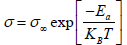(1)

Here σ is the electrical conductivity (S/m) where (S) means Siemens which equal (ohm-1), σ is the maximum electrical conductivity which is at infinite temperature, Ea is the activation energy for electrical conduction which means the threshold energy that the reactants must acquire before reaching the transition state, KB is Boltzman constant and T is the temperature in Kelvin. The term (KBT) represents the average kinetic energy of the molecules and it is obvious that with higher temperature, the kinetic energy will be higher and thus speed up the reaction .

Molar electrical conductivity and walden rule

Molar electrical conductivity is a physical property of a solution and depends on the number of ions that exist in solution . The molar conductivity (?m) in (S.m2/mole) can be expressed in the following formula :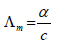(2)

Where c is the molar concentration in (mole/L) or molarity. Walden rule is a relation between the viscosity (?( and the molar conductivity (?m) of the binary solution which can be useful in classifying the ionic liquid solutions and gives a good description about the dissociation of the solvent in the solute. It is summarized in the following formula [7,8]:

?m ηα = m K (3)

Where α is the decoupling constant of the ions 0<α<1 and K (Pose. μS.cm2/mole) is temperature-dependent constant. An ideal line was suggested by Walden which has a slope equal to 1 to classify the ionic liquids into “good ionic” or “poor ionic” as displayed in Figure 1 .

#### Experimental Apparatus

Samples of binary liquid mixtures of water and phenol at different concentrations from 0.00% to 100.00% by weight of phenol were prepared. The viscosity of the samples was measured by using a glass capillary U-tube viscometer. The density of the pure components and the binary mixture was measured by using the Pycnometer. The temperature of the sample was adjusted and controlled by using the Fried Electric model WB-23. A Bante 520 Portable conductivity meter with an accuracy ± 0.5% was used to measure the electrical conductivity of the binary mixture.

#### Results and Calculations

Critical point

Experimental data of dynamic viscosity were measured for water - phenol binary liquid mixture for the concentrations (0.00%, 10.17%, 20.80%, 30.20%, 31.80%, 32.90%, 33.90%, 35.00%, 37.00%, 40.00%, 50.00%, 60.00%, 70.00%, 80.00%, 90.00% and 100.00%) by weight of phenol and temperatures range (32.0ºC -75.0ºC). The dynamic viscosity versus temperature was plotted at different concentrations for the binary liquid mixture of water-phenol in Figure 2.

Figure 2: The dynamic viscosity of water- phenol as a function of temperature for the concentrations (0.00%-35.00%) by weight of phenol.

The dynamic viscosity values are observed to decrease with increasing the temperature from Figure 2. Anomaly in the behavior of the binary mixture is observed at T=67.0 and 33.90% by weight of phenol. This point is the critical point for the mixture where the two liquids become in one phase and completely miscible which was observed by eye. The binary mixture of water-phenol shows anomaly in its behavior at different points. The anomaly at these points was observed because there were some sources of error in the experiment such as the temperature was not adjusted very well or some bubbles of air were formed in the binary mixture which affected the value of the dynamic viscosity.

Electrical conductivity measurements

The electrical conductivity of water - phenol mixture was studied for wide range of temperatures (32.0-75.0°C) and concentrations (0.00% - 100.00%) by weight of phenol. The electrical conductivity measurements as a function of temperature are plotted in Figure 3.

Figure 3: The electrical conductivity versus the temperature for concentrations range (10.17%-37.00%) by weight of phenol.

For each given concentration, the electrical conductivity was observed to increase with temperature. The increment in values of electrical conductivity is due to the increase in speed of ions of waterphenol binary mixture with increasing temperature.

Electrical conductivity values as a function of temperature obeys Arhenius equation which is given by the following formula:-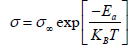(4)

The concentrations (0.00%, 10.17%, 20.80%, 30.20%, 31.80%, 35.00%, 37.00%, 40.00%, 50.00%, 60.00%, 70.00%, 80.00%, 90.00% and 100.00%) except the critical concentration XC=33.90% gives a good agreement with Arhenius equation . The natural logarithm of the electrical conductivity for X=10.17% versus the reciprocal of the temperature are plotted in Figure 4. Arhenius equation gives a good agreement with our experimental values. Slope of Figure 4 representsvalue for each concentration, and intercept with the y-axis represents the natural logarithm for the maximum value of the electrical conductivity lnσ.

Figure 4: The natural logarithm of the electrical conductivity versus thereciprocal of temperature at concentration 20.80% by weight of phenol.

Behavior of electrical conductivity above the critical point

Behavior of electrical conductivity above the critical point was proposed above the critical point to fit our measurements. The electrical conductivity above the critical temperature is fitted by using power law:-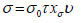(5)

Which can be written in the following formula:-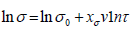(6)

Where τ is the reduced temperature, which is given by:-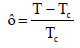(7)

υ is critical exponent=0.64, χσυ is critical exponent=0.037 and σ0 is the noncritical part of the measured electrical conductivity which represents the electrical conductivity at inifinite temperature [10-17].

The electrical conductivity measurements for the critical concentration (XC=33.90%) and above the critical temperature (TC=67.80°C) are listed in Table 1. Eq. (5) can be compared with the result of Figure 5, the value of the critical exponent constant is presented by slope of the curve which equal to 0.0321. The critical exponent χσυ has a good agreement with D’Arrigo and Klein values which equal 0.037 [10,11]. The intercept of the curve represented the natural logarithm of the noncritical part of the measured electrical conductivity lnσo= 3.4098, so the value of the noncritical part equal to 30.26 μS/cm.

T(°C) σ(μS/cm) T-TcLnσ Lnτ
68.0 25.05 0.2 0.0030 3.2209 -5.8260
68.2 25.66 0.4 0.0059 3.2449 -5.1329
68.4 26.03 0.6 0.0089 3.2591 -4.7274
68.6 26.29 0.8 0.0118 3.2691 -4.4397
68.8 26.49 1.0 0.0148 3.2769 -4.2166
69.0 26.66 1.2 0.0177 3.2833 -4.0342
69.2 26.67 1.4 0.0207 3.2835 -3.8801
69.4 26.79 1.6 0.0236 3.2881 -3.7466
69.6 26.90 1.8 0.0266 3.2922 -3.6288
69.8 27.00 2.0 0.0295 3.2959 -3.5234
70.0 27.09 2.2 0.0325 3.2992 -3.4281

Table 1: The electrical conductivity measurements for the critical concentration (XC=33.90%) for water-phenol binary mixture above the critical temperature.

Calculations of molar electrical conductivity

The concentrations of ions in the binary mixture are studied by calculating the molar electrical conductivity and applying Walden’s rule. The molar electrical conductivity was calculated for the concentrations (10.17%, 20.80%, 30.20%, 31.80%, 33.90%, 35.00%, 37.00%, 40.00%, 50.00% and 60.00%) by the weight of phenol. The concentrations of the ions in the binary mixture were studied by applying Walden rule. The logarithm of molar electrical conductivity and the logarithm of the reciprocal of dynamic viscosity at several concentrations at T=68.0°C is drawn as shown in Figure 6.

Figure 6: The logarithm of molar electrical conductivity versus the logarithm of reciprocal of the dynamic viscosity at T=68.0°C.

It can be noticed from Figure 6 that the slope of the Figure 6 α=0.9439 which is lower than 1 and the calculated points for the waterphenol binary mixture lie below the ideal line. This means that waterphenol mixture can be considered as poor ionic solution.

#### Conclusion

The dynamic viscosity was measured for water-phenol binary mixture at several concentrations and temperatures to determine the critical concentration and critical temperature. The critical concentration is determined at 33.90% by weight of phenol and the critical temperature was 67.0°C from the dynamic viscosity measurements and from the electrical conductivity measurements it showed that the critical temperature 67.8°C.

Wilcox and bailey found the critical concentration and critical temperature and it was (36.50% of phenol and 65.3°C). The difference between their results and our results is due to the difference in the purity of the water and phenol which are used in the experiment .

The electrical conductivity measurements are recorded for waterphenol binary mixture for concentration ranges (0.00-100.00%) by weight of phenol and temperature range from 32.0°C to 75.0°C. As the temperature values increase, the electrical conductivity values are observed to increase because the speed of collisions among the species of the mixture increased.

The electrical conductivity measurements at different concentrations are fitted by Arhenius equation.

The activation energy and the electrical conductivity at infinite temperature are calculated for various concentrations. The electrical conductivity is fitted by using power law above the critical temperature and for the critical concentration. The noncritical part of the electrical conductivity is found to be 30.36 μS/cm and the critical exponent was 0.0321.

The ionic properties of the binary mixture is studied by calculating the molar electrical conductivity and then Walden rule is applied to give a relation between the molar electrical conductivity and the shear viscosity of the water-phenol mixture. It is observed from Walden rule, that the slope of the line is 0.9439 which is lower than one. These results mean that the water-phenol mixture has low concentrations of ions which carry the current and can be considered as a poor ionic liquid. The main results of this work and other works are summarized in Table 2.

Parameters This work Previous works
TC 67.0°C (from dynamic viscosity measurements)
67.8°C (from electrical conductivity measurements)
65.3°C
XC 33.9% 36.50%
Ea 4.0128(J ×10-20) at (0.00%)
5.1325(J ×10-20) at (10.17%)
6.0481(J ×10-20) at (20.18%)
6.4842(J ×10-20) at (50.00%)
-
σ 151448.40µS/cm at (0.00%)
3355126.00µS/cm at (10.17%)
26429728.00µS/cm at (20.18%)
29151007.00µS/cm at (50.00%)
-
χσυ 0.0321 0.037
0.037 
σo 30.26µS/cm
26.85 µS/cm
-
α 0.9439 -

Table 2: The main results of this work and other works.

#### References

Select your language of interest to view the total content in your interested language

### Article Usage

• Total views: 9632
• [From(publication date):
April-2016 - Aug 21, 2019]
• Breakdown by view type
• HTML page views : 9500
• PDF downloads : 132

## Post your commentCan't read the image? click here to refresh
###### Peer Reviewed Journals

Make the best use of Scientific Research and information from our 700 + peer reviewed, Open Access Journals

International Conferences 2019-20

Meet Inspiring Speakers and Experts at our 3000+ Global Annual Meetings

Top